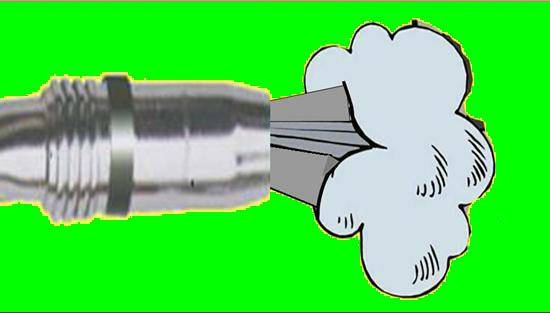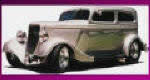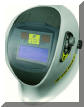This Page Presents Welding 4130 Chrome Moly (Chromoly) Math, and Other Information About Welding 4130: Click for BASIC 4130 WELDING DETAILS Click for TECHNICAL & METALLURGICAL DETAILS Click for METALLURGICAL DEFINITIONS Click for WELDING A BETTER STEEL;  HY 130 Click for DEFINING "EFFECTIVE HEAT INPUT"

Math Used to Generate 4130 Information.

The Information Below;Provides the math equations that estimate the theoretical cooling rates for TIG welds in thin wall 4130 tubes.The cooling rate equations were obtained from the Welding Handbook, Volume 1, 9th Edition, page 100.Both thick and thin plate equations were developed by Professor C.A.  Adams et al.
The data is presented in a way so one can see the numbers from each part of the equation. As the thickness was increased so was the heat input.  A TIG process efficiency of 0.5 (50%) was used which is somewhat  higher than the sited Welding Handbook reference but errors on the side of predicting a slower cooling rate. The thin plate equation is for two dimensional heat flow and may be OK for a butt weld but a Tee fillet weld will have faster cooling rate. Therefore despite trying to error on the side of predicting slower cooling rates, they are all around the 1/4 in Jominy bar location. The exact cooling rate will be somewhat dependent on the diameter of the tube as well. However I would expect that perhaps 1/2 way around the joint the cooling rate would be similar to that predicted. As the complete circular weld is made the last part will have a preheat from the weld start. However if a brittle structure is formed in any area and a crack forms, that is sufficient to be a problem.
Equation Elements and ValuesRc = Cooling rate at weld centerline; deg F/mink = Thermal Conductivity of metal; BTU/min in deg Fp = Density of metal; lb/in3C = Specific Heat of metal; BTU/ (lb deg F)h = thickness of metal; inHnet = Net heat input; BTU/in [V*I / ipm*.057*process efficiency]Tc = Temperature which cooling rate is calculated; deg FTo = Initial plate temperature; deg F

Welding Cooling Equations from page 100 Welding Handbook Volume 1, 9th Edition

Thick Plate Equation =Rc =-[2*3.1416*k(Tc-To)2] / Hnet
Thin Plate Equation = Rc = 2*3.1416*kpC (h/Hnet)2(Tc-To)3

Calculate estimated cooling rate @ 1100 deg F for thin wall 4130 tube welds:

Tube = .040 in wall; V (volts) = 10; I (amps) = 70; travel = 12 ipm

Using thin plate equation:-2*3.1416*0.024*0.29*0.11= -.00481Hnet = 1.66(h/Hnet)2 = 0.000579(Tc-To)3 = 1.07*109Rc = -2981 deg F / min = - 50 deg F / sec
Tube = .0625 in wall; V (volts) = 10; I (amps) = 90; travel = 11 ipm

Using thin plate equation:-2*3.1416*0.024*0.29*0.11= -.00481Hnet = 2.33(h/Hnet)2 = 0.000718(Tc-To)3 = 1.07*109Rc = -3700 deg F / min = - 62 deg F / sec
Tube = .093 in wall; V (volts) = 10; I (amps) = 110; travel = 9 ipm

Using thin plate equation:-2*3.1416*0.024*0.29*0.11= -.00481Hnet = 3.48(h/Hnet)2 = 0.000713(Tc-To)3 = 1.07*109Rc = -3671 deg F / min = - 61 deg F / sec
REFERENCES USED;US Steel Atlas of Isothermal Transformation DiagramsRepublic Alloy steelsR. A. Grange and Kiefer; "Transformation of Austenite on Continuous Cooling and Relation to Transformation at Constant Temperature."Walter Crafts and John Lamont; "Hardenability and Steel Selection."AWS Welding Handbook; Volume 1, 9th AdditionThe "Gas Blast" at MIG Weld Starts Causes Problems:

1) Shielding Gas Waste - typically over half the gas used

2) Inferior Weld Quality - due to air pulled in gas stream

If a Search Engine Found This Page 1st- - - We'd Suggest a Visit to The Basic Welding 4130 Page; Then Return.  Click Here

This page presents the equations that support the data in the 4130 Technical Details page.

PURCHASE GSS PRODUCT

Check Out Welding Math Site

Click for BASIC 4130 WELDING DETAILS

Click for TECHNICAL & METALLURGICAL DETAILS

Click for  EQUATIONS defining weld cooling rate in tubing

Click for METALLURGICAL DEFINITIONS

Click for WELDING A BETTER STEEL;  HY 130Stop Wasting Shielding Gas!!

Including Our Patented MIG Shielding

"Gas Saver System" (GSS)

Rat Rods-Then and Now

Welding: Go Green

Engineering A Pro Street / Street RodENGINE - CLICKTUNING HOLLEY CARB - CLICKENGINE COOLING- CLICKWIRING - CLICKCHASSIS - CLICK"WARNING: "Weld Safely"